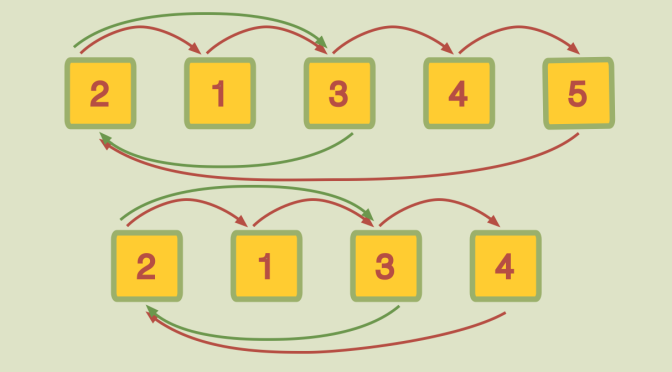Generating the symmetric group with a transposition and a maximal length cycle

Can the symmetric group $$\mathcal{S}_n$$ be generated by any transposition and any $$n$$-cycle for $$n \ge 2$$ integer? is the question we deal with.

We first recall some terminology:

Symmetric group
The symmetric group $$\mathcal{S}_n$$ on a finite set of $$n$$ symbols is the group whose elements are all the permutations of the $$n$$ symbols. We’ll denote by $$\{1,\dots,n\}$$ those $$n$$ symbols.
Cycle
A cycle of length $$k$$ (with $$k \ge 2$$) is a cyclic permutation $$\sigma$$ for which there exists an element $$i \in \{1,\dots,n\}$$ such that $$i, \sigma(i), \sigma^2(i), \dots, \sigma^k(i)=i$$ are the only elements moved by $$\sigma$$. We’ll denote the cycle $$\sigma$$ by $$(s_0 \ s_1 \dots \ s_{k-1})$$ where $$s_0=i, s_1=\sigma(i),\dots,s_{k-1}=\sigma^{k-1}(i)$$.
Transposition
A transposition is a cycle of length $$2$$. We denote below the transposition of elements $$a \neq b$$ by $$(a \ b)$$ or $$\tau_{a,b}$$.

We also recall some theorems on the symmetric group.

Theorem 1: The symmetric group $$\mathcal{S}_n$$ is generated by its transpositions.
Proof.: We induct on $$n$$. The theorem is trivial for $$n=2$$. Now, suppose that $$\sigma$$ is an element of $$\mathcal{S}_{n+1}$$. We consider the permutation $$\sigma^\prime$$ equal to $$\sigma$$ if $$\sigma(n+1)=n+1$$ and to $$\tau \circ \sigma$$ if $$\sigma(n+1)=i \neq n+1$$ where $$\tau$$ is the transposition $$(i \ n+1)$$. In either case: $$\sigma^\prime(n+1)=n+1$$. Let $$\mathcal{S}_{n+1}^\prime$$ be the subgroup of elements of $$\mathcal{S}_{n+1}$$ for which $$n+1$$ is a fixed point. Evidently, $$\mathcal{S}_{n+1}^\prime$$ is isomorphic to $$\mathcal{S}_n$$ by restrictions of mappings. So by inductive hypothesis, $$\sigma^\prime$$ is equal to a product of transpositions. And so is $$\sigma$$ as $$\sigma = \sigma^\prime$$ or $$\sigma=\tau \circ \sigma^\prime$$. This concludes our proof by induction.

Theorem 2: The symmetric group $$\mathcal{S}_n$$ is generated by the transpositions $$(\tau_{i,i+1})_{1 \le i \le n-1}$$.
Proof.: By theorem 1, it is enough to prove that any transposition $$\tau_{a,b}$$ is generated by $$(\tau_{i,i+1})_{1 \le i \le n-1}$$. Without loss of generality, suppose $$a < b$$. We induct on $$b-a \ge 1$$. Our base case, $$b=a+1$$ is trivial. For the inductive step, we note that: $\tau_{a,b}=\tau_{a,a+1} \tau_{a+1,b} \tau_{a,a+1}$ Therefore if $$\tau_{a+1,b}$$ is in the subgroup generated by $$(\tau_{i,i+1})_{1 \le i \le n-1}$$, it follows that $$\tau_{a,b}$$ is, as well. Theorem 3: $$r=(1 \ 2)$$ and $$s=(1 \ 2 \dots \ n)$$ generate $$\mathcal{S}_n$$.
Proof.: By theorem 2, this is a consequence of the relations: $$\tau_{i,i+1}=s^{i-1} \circ r \circ s^{-(i-1)}$$ for $$1 \le i \le n-1$$.

Returning to our initial question, we prove that when $$n$$ is a prime number $$p$$, then any transposition and any $$p$$-cycle generate $$\mathcal{S}_p$$.
Proof.: Let
$(a \ b \dots c \ d) \text{ and } (e \ f)$ be any $$p$$-cycle and any transposition. Any automorphism of $$\{1, \dots, p\}$$ yields an automorphism of $$\mathcal{S}_p$$ (more precisely an inner automorphism). Hence we can rename $$e=1$$ such that the permutations are now:
$(a^\prime \ b^\prime \dots 1 \dots c^\prime \ d^\prime)=(1 \dots c^\prime \ d^\prime \ a^\prime \ b^\prime \dots) \text{ and } (1 \ f^\prime)$ Now, for some $$1 \le k \le p-1$$:
$(1 \dots c^\prime \ d^\prime \ a^\prime \ b^\prime \dots)^k=(1 \ f^\prime \dots)$ and as $$p$$ is supposed to be prime, $$(1 \ f^\prime \dots)$$ is a $$p$$-cycle. We can again rename the elements of $$\{1, \dots, p\}$$ such that $$f^\prime = 2$$ and the rest accordingly to get:
$(1 \ 2 \dots p-1 \ p) \text{ and } (1 \ 2)$ for the $$p$$-cycle and the transposition that are our predefined generators. According to theorem 3, those permutations generate $$\mathcal{S}_n$$.

We finally show a counterexample. For instance $$(1 \ 3)$$ and $$(1 \ 2 \ 3 \ 4)$$ do not generate $$\mathcal{S}_4$$. The reason is that these two permutations preserve congruences modulo $$2$$ (if two numbers in $$\{1, 2, 3, 4\}$$ are both even or both odd, applying either permutation to them returns values that are both even or both odd), so the subgroup they generate in $$\mathcal{S}_4$$ has this property while $$\mathcal{S}_4$$ does not: $$1,3$$ are both odd, while their images by the transposition $$(1 \ 2)$$ have different parities.